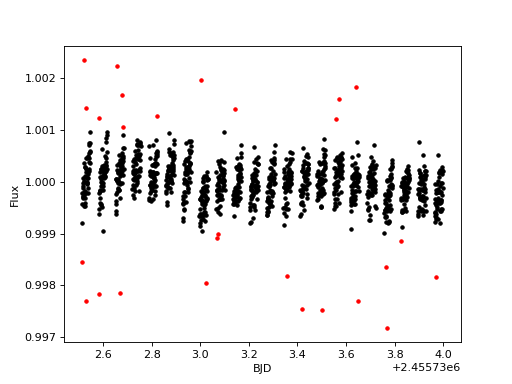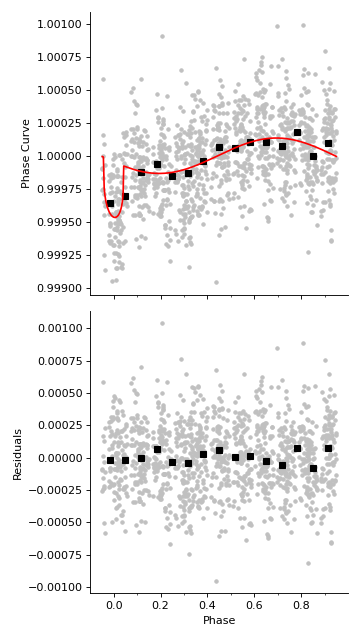# Phase Curve of 55 Cnc e¶

## Introduction¶

Warning

This tutorial does not use real CHEOPS data, only a realistic example data set. Don’t interpret any science results from this example!

55 Cnc e is perhaps the rocky planet most amenable to characterization with CHEOPS. This super-Earth with radius 1.91 Earth radii and mass 8.08 Earth masses orbits a very bright (V = 6) G8V host star in a 17 hour orbit (Winn et al. 2011; Demory et al. 2016b). There is evidence for two distinct plausible climatic scenarios for 55 Cnc e: either it is a lava world without a significant atmosphere (Demory et al. 2016a), or it has a very thick atmosphere (Angelo & Hu 2017). Existing transit observations of 55 Cnc e at 3.6 and 4.5 microns show similar transit depths, also consistent with an opaque atmosphere or no atmosphere (Zhang et al. in prep.).

55 Cnc e is also a touchstone system among ultra-short period rocky planets, a class of objects which may be uncovered by the TESS mission in the near future. Ultra-short period rocky planets may not have been born that way. A growing body of evidence suggests that some ultra-short period planets may have once been giants that have lost part of their gaseous envelopes due to photoevaporation (Fulton et al. 2017; Van Eylen et al. 2018). 55 Cnc e may represent one end-point of planetary evolution under high irradiation.

CHEOPS observed 55 Cnc on May 23, 2020 UTC for 26 hours. This was the first of many visits planned on 55 Cnc. In this tutorial, we’ll demonstrate how to reduce a convincing simulated example dataset similar to the first CHEOPS observations of 55 Cnc e in order to recover the phase curve signal from the exoplanet.

## Sigma clipping¶

First, let’s import some packages we’ll need, including linea:

import numpy as np
import matplotlib.pyplot as plt
from scipy.optimize import fmin_l_bfgs_b
from batman import TransitModel

from linea import CheopsLightCurve, Planet

# Load the transit parameters for 55 Cnc e
p = Planet.from_name('55 Cnc e')

# Load the example light curve of 55 Cnc e (built into the package)
lc = CheopsLightCurve.from_example()


In the last line, we initialized a CheopsLightCurve object using the from_example method, which loads example (fake) data of 55 Cnc e.

Then we sigma-clip the light curve – this removes outliers.

# Sigma clip the light curve to remove outliers
lc.sigma_clip_flux(sigma_upper=3, sigma_lower=3, plot=True)The red points are masked from further calculations, black points are included in the remaining analysis.

## Fitting for the transit time¶

Now let’s fit for the transit time, using scipy to minimize the $$\chi^2$$. First we must define the function to minimize, then let’s call fmin_l_bfgs_b to minimize the $$\chi^2$$ by varying the transit time:

def chi2(theta):
"""
Fit for the best transit time
"""
t0, = theta
transit_model = TransitModel(p, lc.bjd_time[~lc.mask] - t0,
supersample_factor=3,
exp_time=lc.bjd_time - lc.bjd_time,
).light_curve(p) - 1

# Build a custom design matrix with a transit model plus the defaults
X = np.hstack([
# Transit model:
transit_model[:, None],

# Default design matrix:
lc.design_matrix(),
])

# Least squares regression:
r = lc.regress(X)

# Return the chi^2
return np.sum((lc.flux[~lc.mask] - r.best_fit)**2 /

initp = [0.0]

# Minimize the chi^2
t0_offset = fmin_l_bfgs_b(chi2, initp, approx_grad=True, bounds=[[-0.1, 0.1]])


## Regression analysis¶

Now we have the transit time offset, we can compute the transit model that we’ll use in the regression analysis, which we’ll call transit_model_offset.

# Compute a transit model with the time offset we found previously
transit_model_offset = TransitModel(p, lc.bjd_time[~lc.mask] - t0_offset,
supersample_factor=3,
exp_time=lc.bjd_time - lc.bjd_time,
).light_curve(p) - 1


Next we need to build a design matrix, which contains column vectors of the observational variables which we wish to detrend the flux against. Most of these column vectors are built into the CheopsLightCurve object, available under the design_matrix method. The additional vectors we add to our design matrix $$X$$ are the transit model, and a sinusoidal trend, which will represent the phase curve variations of 55 Cnc e.

delta_t = lc.bjd_time[~lc.mask] - lc.bjd_time.mean()

# Build a design matrix
X = np.hstack([
# Transit model:
transit_model_offset[:, None],

# Sinusoidal phase curve trend:
np.sin(2 * np.pi * delta_t / p.per)[:, None],
np.cos(2 * np.pi * delta_t / p.per)[:, None],

# Default design matrix:
lc.design_matrix(),
])


To do the linear regression, simply call the regress method:

r = lc.regress(X)


The solution to the linear regression is stored in r. Now we can set up some parameters which will be necessary for plotting the phase curve, namely a transit model and sinusoidal trend which span the full time interval:

t_fine = np.linspace(lc.bjd_time.min(), lc.bjd_time.max(), 1000)
delta_t_fine = t_fine - t_fine.mean()

transit_fine = TransitModel(p, t_fine - t0_offset,
supersample_factor=3,
exp_time=lc.bjd_time - lc.bjd_time,
).light_curve(p)

sinusoid_fine = np.hstack([
np.sin(2 * np.pi * delta_t_fine / p.per)[:, None],
np.cos(2 * np.pi * delta_t_fine / p.per)[:, None],
]) @ r.betas[1:3]


Finally let’s call the plot_phase_curve method to plot the phase curve, with the best transit and sinusoidal models:

fig, ax = lc.plot_phase_curve(r, p, t_fine, transit_fine, sinusoid_fine)Note

The above plot is a simulated example light curve, not real CHEOPS observations. Do not make any conclusions about the planet from this fake dataset.

The transit (when the planet occults the host star) occurs near phase zero. The planet’s orbital phase is normalized such that an entire orbit spans the range zero to one, with the secondary eclipse near 0.5 (not significantly detected in this single visit). The upper panel shows the detrended CHEOPS observations (gray), the binned CHEOPS observations (black), and the expected signal from the transit and the phase curve combined (red). The lower panel shows the residuals, or the CHEOPS observations with the best-fit transit and phase curve model subtracted.